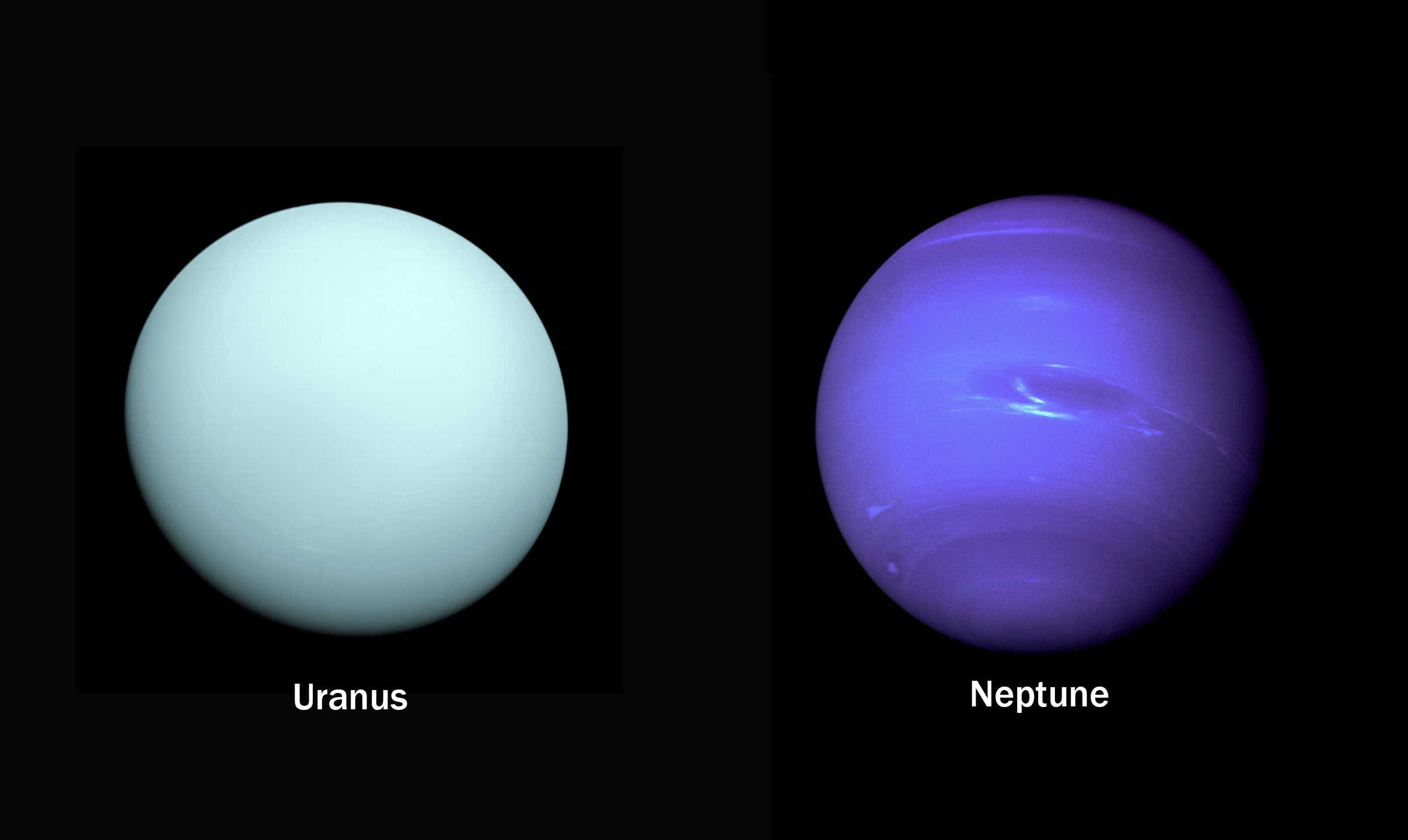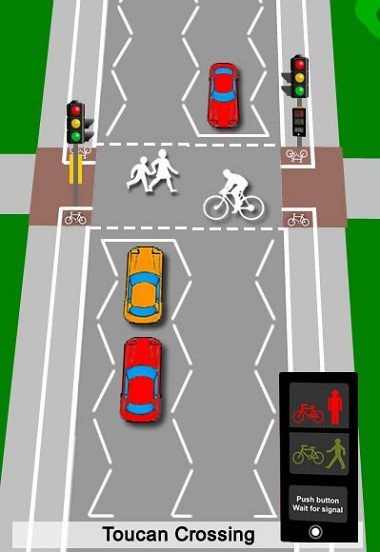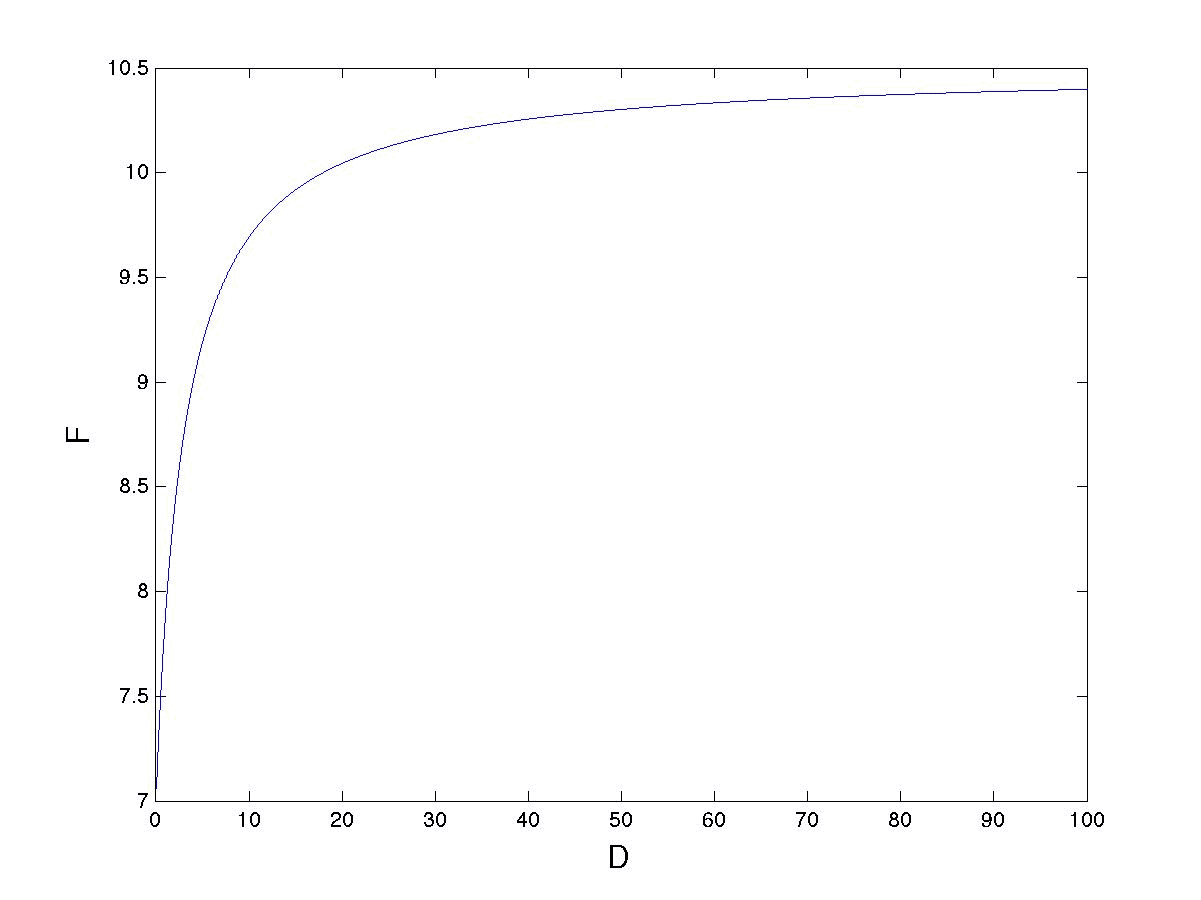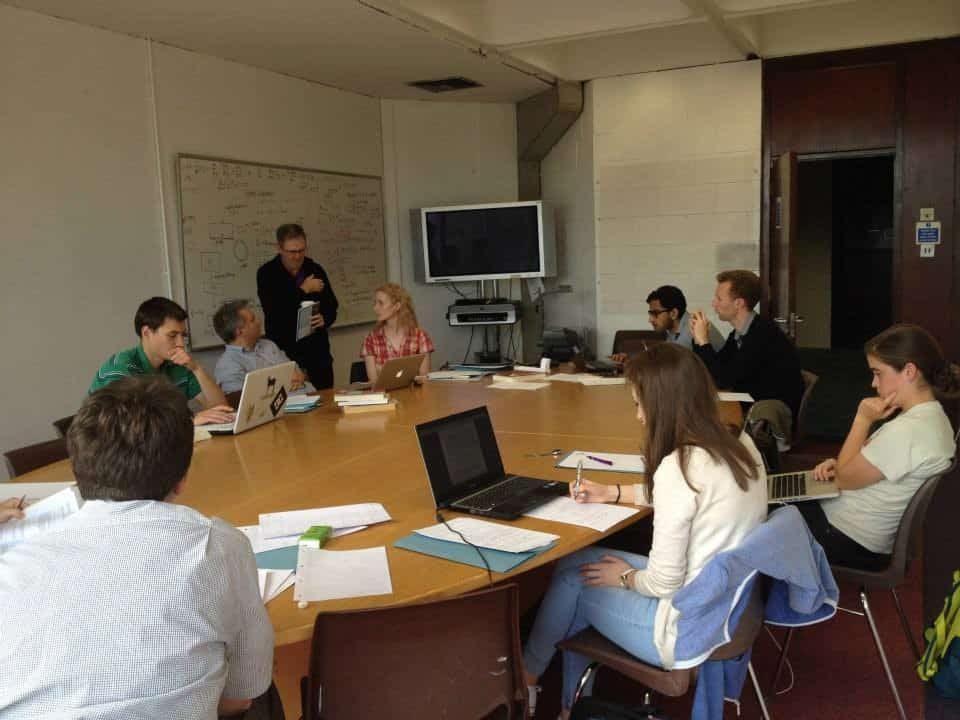Mathematical Modelling impacts every aspect of our lives, and here I will look at a series of real world examples which show just how important it is. This article follows on from another article where I looked at the more general question of ‘What is Mathematical Modelling’?

## Physical Sciences and Engineering

These often start from quite well-known laws, and are then simplified. This is most often done by working out the size of different effects, and then ignoring those which are considered too small to make a big difference to the final result. This can either be done mathematically, or by experiments. Sometimes this is combined with assumptions about how the system will behave to simplify the number of possibilities that need to be studied.

It is worth considering two examples, which show the real predictive power of this process. The first comes from celestial mechanics. After Newton had written down the laws of motion for the solar system they were found to be very accurate in predicting the paths of the then known planets. However, on 13th March 1781 the planet Uranus was discovered by William Herschel. When its orbit was plotted it was found that whilst it nearly agreed with the predictions of Newtonian mechanics, there were small discrepancies. At this stage the trust in the accuracy of the underlying model was such that even these small discrepancies caused a large amount of concern. (This would not happen with a model from biology or the social sciences!)

It was postulated that there must be a cause, and one explanation was that there was another planet, which was disturbing the orbit of Uranus. Using the Newtonian model for the solar system it was possible to calculate the location of this planet. This calculation was carried out independently, by John Couch Adams at Cambridge, and by Urbain Le Verrier in Paris. Both obtained very similar answers, and in response to Le Verrier’s calculations, Johan Galle discovered the planet in 1846. We now call this planet Neptune, and it is illustrated below with Uranus on the left.The key lesson from this example was that a mathematical model was able to predict something completely new, which was not built into the model in the first place. It became clear at this point that mathematical models had potentially extraordinary predictive powers.

The second example comes from climate science. In general, climate models (or really simulations) are far too complex to be used to make analytical predictions. The best that we can do is to run them many times on different scenarios of, for example, Carbon Dioxide production and solar variability. However, a simple climate model which assumes that the energy arriving from the Sun balances the energy radiated from the Earth gives us an equation for the average temperature of the Earth T in formHere S(t) is the average energy from the Sun, a is the albedo of the Earth, e is the effective emissivity of the Earth’s atmosphere and sigma is Boltzman’s constant. This is a true model. It is a complete simplification of a very complex system. However it is predictive, and allows us to see how the temperature of the Earth will change if the energy from the Sun changes (varying S), the polar ice caps melt (varying a) or if Carbon Dioxide changes (varying e). It is also a good model in the sense that these predictions can be tested against experimental data.

## Biological Sciences

It has taken longer for mathematical modelling to make an impact in the biological sciences. This is mainly because biological systems are inherently much more complex than physical ones (consider modelling a cell or the brain for example). It is also generally harder to make, repeat, and quantitatively assess, experiments on biological systems. However, by a lot of careful study, significant progress has been made in recent years, starting with pioneering work by Alan Turing just after the Second World War. Mathematical models are now used to help understand changing animal populations, the evolution of biological patterns, the spread of disease and the functioning of the nervous system.

## Social Sciences

Modelling is both useful and important here, but is complicated by the fact that we have to take human behaviour into account. Given the complexities of the way that humans think and behave, this is generally hard, if not impossible. However, the mathematics of game theory can be used to describe how economies behave, and how competition and (possibly) altruism can evolve.

## The Rest

In my career I have been asked to model many things, including the reaction of homeless people to changes in government policy, microwave cooking, fish in an aquarium, organ playing, cocoa growing, and the transportation of biscuits in a factory. These I all take seriously and do my best. However I draw the line at questions (usually posed by journalists when there is not much news around) such as what is the ideal shape for a Christmas tree, what is the perfect kiss, and what is the perfect joke? The key advice in all such cases is: avoid the question. The journalist is not looking for an answer, instead they are looking for a way to make fun of the person trying to give the answer. Avoid!

## How Did the Mathematician Cross the Road?

You will all be familiar with crossings where you press a button and wait for the pedestrian light to go green, accompanied by a lot of beeping. I’m sure that you will all have noticed that when you press the button there is a delay D before the light goes green. The reason for this delay is to make sure that the traffic isn’t blocked by a constant flow of pedestrians. But the question is: how long should the delay be? Too short and the traffic is blocked. Too long and the pedestrians give up waiting and chance it by running across the road. To answer this question we can have a go at using a simple mathematical model for the crossing process.

We start by thinking about what the ingredients of the model. A picture always helpsA simple set of initial assumptions are as follows:

• Pedestrians arrive at a steady rate at the crossing
• Traffic arrives at a steady rate
• It takes the pedestrians a time T to cross the road
• The ideal delay time is the one that maximises the average total flow F of both the pedestrians and the traffic.

By a steady rate we will assume that in any time interval of length t, thenpedestrians will arrive andcars will arrive. We will also assume that all of the pedestrians waiting at the lights cross at once when the lights turn green.

Once the lights go red again we will wait a time 1/a before a pedestrian arrives and presses the button to cross. The lights then have a delay D during which cars can cross before they go again.

Now to do some maths. We have a complete cycle of time 1/a + D+T. In this time 1 + a*D pedestrians arrive and then cross. In the same time b*(1/a +D) cars cross over the crossing.

The average total flow of pedestrians and cars is then given by:If we take (for example) a = ½, b = 10, T = 1 then a plot of F as a function of D is given below:We can see from this model that the total flux increases as D increases. Indeed it rises from

F = (a+b)/(1 + aT) when D = 0, to F = (a+b) as D tends to infinity.

We conclude from this simple model that it is best to make the delay D as long as possible. However there will be an upper limit. This depends on the patience of the pedestrians. This is beyond the subject of mathematical modelling.

Readers of these notes might like to consider whether they agree with this model, the assumptions made to get it, or the conclusions that we have drawn from it. Indeed, how might the model be improved, and does it affect the conclusion? For example, is the assumption that the best delay time is one which maximises the flow, or is it better to minimise the average waiting time of the pedestrians/cars? I leave these as a matter of discussion.

# Common Mistakes in Mathematical Modelling

All models, even complex simulations, are approximations of reality. They are not reality itself. This should always be born in mind when making predictions. Far better to say ‘that my model for climate change predicts a rise of three degrees in temperature’, than to say that there will be a three degree rise. When using a model always be aware of the limitations, uncertainties and approximations that go into them. The best models have a quantification of their uncertainty.

## Wishful Thinking

It is a very common mistake in mathematics (and indeed in most things) to change a problem to something that we can solve, rather than solving the original problem because it is too hard. A side effect of this is that we might end up using overly sophisticated maths (or indeed the wrong sort of maths altogether) to solve what is in effect the wrong problem.

## The Curse of the Formula

The examples of models that I have shown above have led to simple formulae. But don’t assume that this will always be the case, and certainly don’t over simplify the problem to get to an exact formula.

# Mechanisms for Teaching and Learning Mathematical Modelling

Because mathematical modelling is so important, it is essential that it is taught, and that it is taught well. Students respond very well to seeing how mathematics can have applications that they never thought were possible. It is good to see that some mathematical modelling is now taught in both A level and undergraduate mathematics courses. By far the best way to teach it is to immerse the students directly in the problem solving environment, and to put them into teams to do this. Even better if the problems come directly from industry. One mechanism to do this, which has proved effective for over 50 years, is the study group model. In these week long workshops, industrial problems are presented on the first day, and the participants work on them in teams, to present a paper/talk on the final day. The work done during the week is then followed up with longer interactions with the company. This mechanism is very powerful, not only for solving the problems (using mathematics), but in training the participants on all sides, and introducing them to new ideas and methods. Originally developed in the UK the study group model is now used all over the world and has proved its value . Below we see a typical study group team in action at a recent workshop in Ireland. Note the very full whiteboard in the background.

###References

 M. Barons, C. Budd, M. Butchers and J. Jordan, Study groups, what are their value?, Mathematics Today, December, (2019).

### Further Material

What Is Mathematical Modelling?

Pythagoras Saves the Whales

Full 58 minute Gresham Lecture on YouTube – Can Maths Save The Whales and Cure Cancer? By Chris Budd

Photo by NASA on Unsplash

By Professor Chris Budd OBE, University of Bath

Featured Image – Photo by Guillermo Ferla on Unsplash